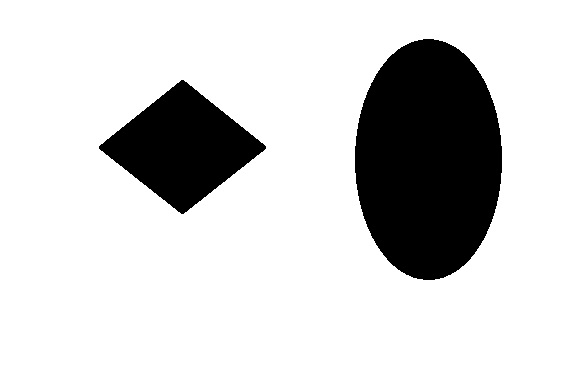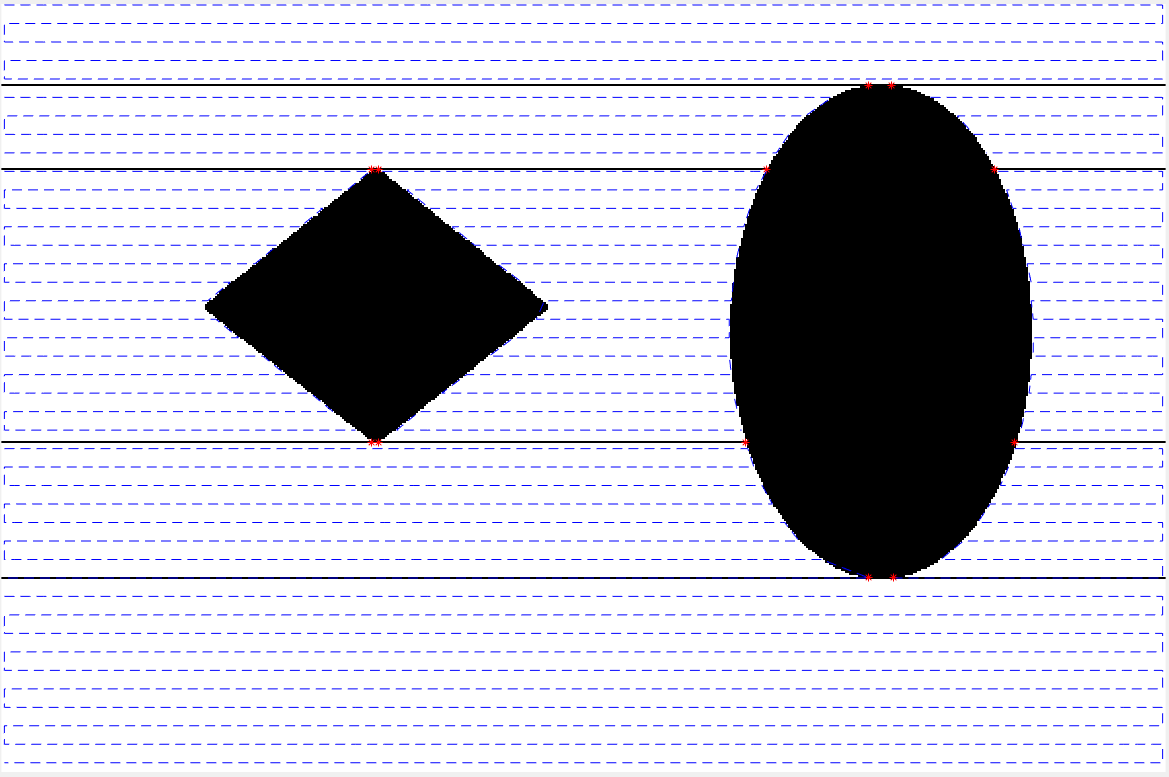# Boustrophedon Decomposition

## Applications:

Automated Lawn-mowers and Vacuum Cleaners.

Matlab Simulation Code Repository:

#### https://bitbucket.org/srikanthmalla/boustrophedon_decomposition

Input:Output:```  			function [path,A]=boustrophedon(polygon_image)
%% intro
%boustrophedon means moving left to right and right to left
%give any irregular polygon then it decomposes into sub poygons by finding
% the critical points
%input- image of irregular polygon
%output- coverage path

% there should be continuity in the free space (which will make
% connectivity between subcells
%% code
A= imread(polygon_image);
A=im2bw(A);
x=zeros(size(A,1),1);
idy=[];obstacle_points=[];critical_points=[];
for i=1:size(A,1)
[x(i),idy]=no_of_lines(A(i,:));
obstacle_points=[obstacle_points;[i*ones(size(idy,1),1),idy]];
end
[idx,no_of_cells]=find_boundary_ids(x,A)
for i=1:size(idx,1)
critical_points=[critical_points;obstacle_points(find(idx(i)==(obstacle_points(:,1))),:)];
end
dist=9;
path=make_path(A,x,dist,no_of_cells,idx);
A(idx,:)=0;
% A=create_boundaries(A,critical_points);
imshow(A);
hold on;
plot(critical_points(:,2),critical_points(:,1),'r*');

for i=1:no_of_cells
plot(path{i}(:,1),path{i}(:,2),'b--');
end
end
```